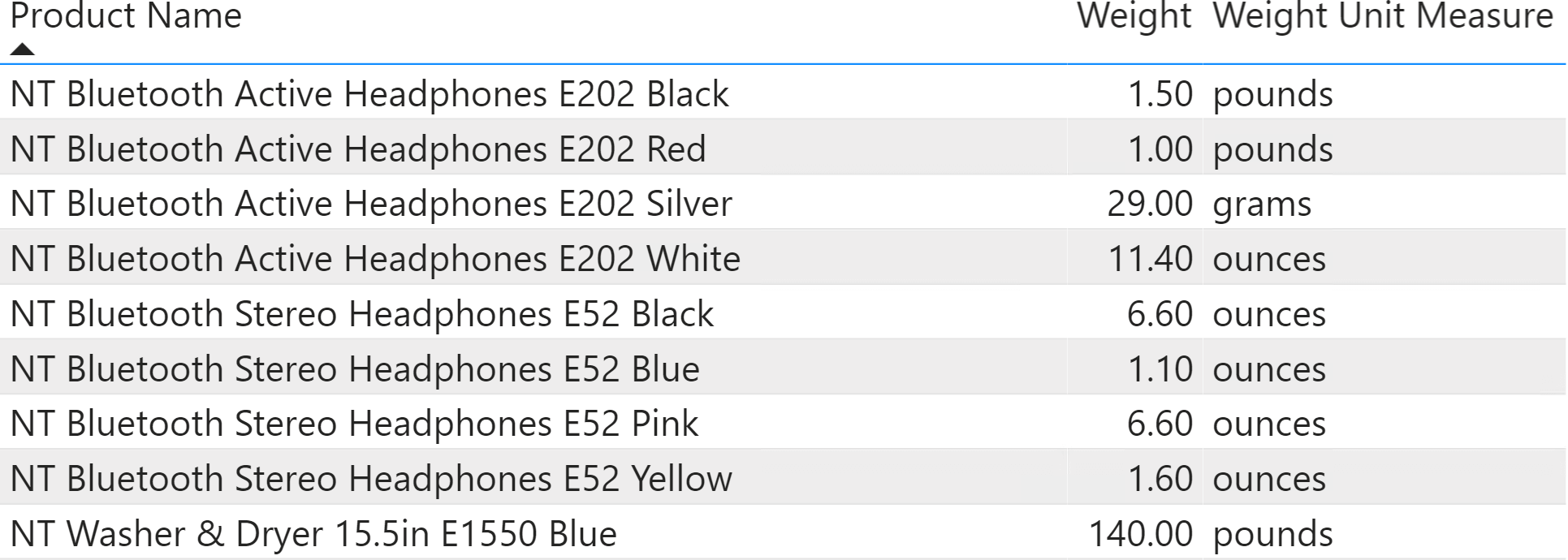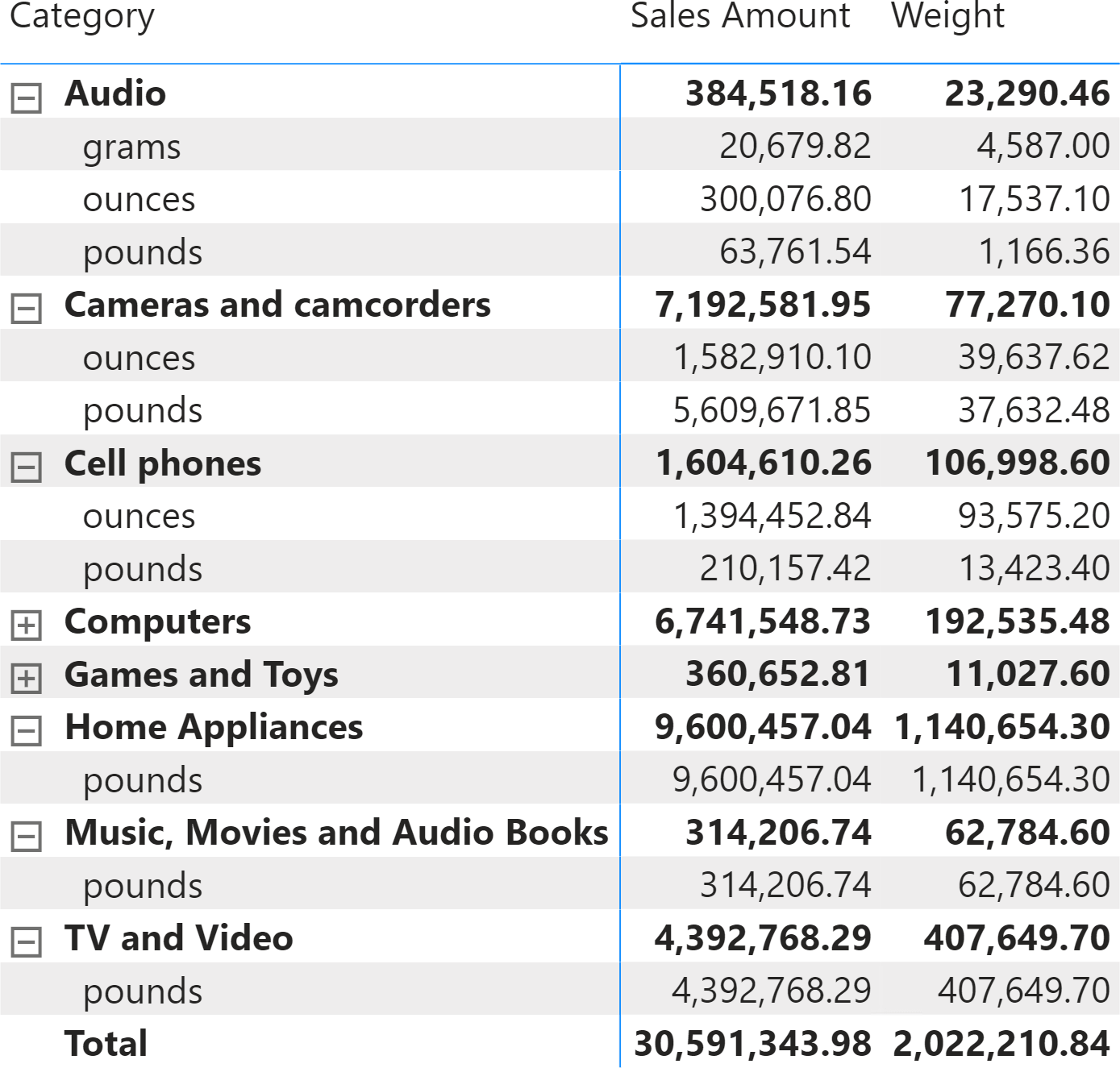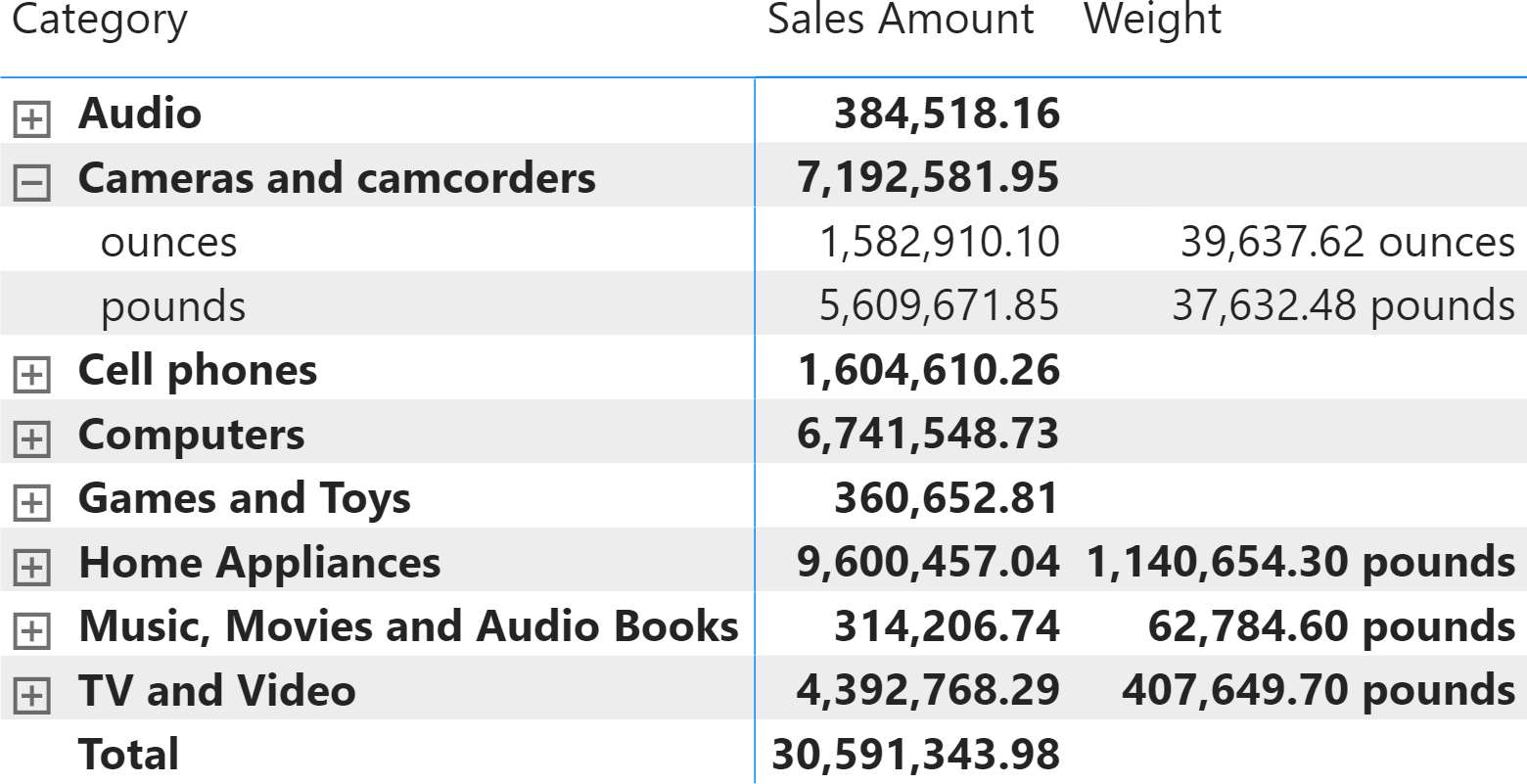Each product in Contoso weighs a certain weight. The weight is stored in two columns: the unit of measure and the actual weight, expressed in that unit of measure. Specifically, Contoso uses three units of measure: ounces, pounds, and grams.Because the units of measure are different, you cannot aggregate the weight over different products. If you author a simple measure that computes the ordered weight of products by using a simple SUMX, the result is wrong:

```Weight := SUMX ( Sales, Sales[Quantity] * RELATED ( 'Product'[Weight] ) )
```

This measure, projected in a matrix, produces a meaningless total because it is aggregating different units of measure.We want to author a new measure that performs two operations:

• It does not show a result if it is aggregating values with different units of measure;
• It adds the unit of measure to the result, as part of the format string.

The result we want to obtain is the following.The first operation is simple, because it is enough to check that there is only one value for the unit of measure visible in the current filter context. The second operation, adding the unit of measure to the format string, is more complex. Indeed, the format string must change depending on the context. Regular measures do not have the option of using dynamic format strings.

That said, dynamic format strings are available in calculation groups. Therefore, we create a calculation group to be able to use the dynamic format strings of the calculation group as an additional feature of our regular measure. In order to make the report work, it is necessary to add a filter to the calculation group. This can be obtained by adding a page-level filter to the report, so that the filter is active without wasting real estate on the screen.

The calculation group is named WeightFormatString, and it has two calculation items named No conversion and Apply format string:

```CALCULATIONITEM WeightFormatString[WeightFormatString]."No conversion" =
SELECTEDMEASURE ()

CALCULATIONITEM WeightFormatString[WeightFormatString]."Apply format string"=
IF (
ISSELECTEDMEASURE ( [Weight] ),
IF (
HASONEVALUE ( 'Product'[Weight Unit Measure] ),
SELECTEDMEASURE ()
),
SELECTEDMEASURE ()
)
FORMAT_STRING =
IF (
ISSELECTEDMEASURE ( [Weight] ),
VAR CurrentFormatString =
COALESCE ( SELECTEDMEASUREFORMATSTRING (), "#,#.00" )
VAR CurrentUnitOfMeasure =
SELECTEDVALUE ( 'Product'[Weight Unit Measure] )
VAR Result =
IF (
NOT ISBLANK ( CurrentUnitOfMeasure ),
CurrentFormatString & " " & CurrentUnitOfMeasure
)
RETURN
Result,
SELECTEDMEASUREFORMATSTRING ()
)
```

Both the calculation item and the format string are applied only to the Weight measure. If there is only one value active in the filter context for the Weight Unit Measure column, then the format string expression is updated by appending the unit of measure to the original format string.

Now that we have created the calculation group, we can actually use it to perform something better. The idea is to let the user choose a reporting unit of measure and perform the conversion of the weight of products on the fly, from their own unit of measure to the required one.

By doing this, we can show totals too. Indeed, any weight is converted into a single unit of measure, and the result can now be aggregated.

To perform this, we added three calculation items: Ounces, Pounds, and Grams. Here we show the code for Ounces. The other two calculation items are very similar, and you can check this by downloading the demo file:

```CALCULATIONITEM WeightFormatString[WeightFormatString]."Ounces" =
IF (
ISSELECTEDMEASURE ( [Weight] ),
VAR ConversionToOunces =
DATATABLE (
"@Unit Measure", STRING,
"@Factor", DOUBLE,
{
{ "ounces", 1 },
{ "pounds", 16 },
{ "grams", 0.035274 }
}
)
VAR Weights =
VALUES ( 'Product'[Weight Unit Measure] ),
"@Unit Measure", 'Product'[Weight Unit Measure] & "",
"@Weight", SELECTEDMEASURE ()
)
VAR WeightsWithFactors =
NATURALLEFTOUTERJOIN ( Weights, ConversionToOunces )
VAR Result =
SUMX ( WeightsWithFactors, [@Factor] * [@Weight] )
RETURN
Result,
SELECTEDMEASURE ()
)

FORMAT_STRING =
IF (
ISSELECTEDMEASURE ( [Weight] ),
SELECTEDMEASUREFORMATSTRING () & " ounces",
SELECTEDMEASUREFORMATSTRING ()
)
```

The code is interesting to analyze. It first creates a table with the conversion factor from the source units of measure to ounces. It then computes in a temporary table the weights, still in their original unit of measure. Finally, it uses NATURALLEFTOUTERJOIN to merge the two tables so that the resulting variable (WeightsWithFactors) includes both the original weight and the conversion factor. The last step is a simple iteration over WeightsWithFactors that sums the partial multiplications.

The resulting model has a calculation group that lets the user choose the desired output and performs the conversion on the fly.As you can see, using calculation groups opens up more modeling options – such as the implementation of dynamic format strings for specific measures. Besides, their real power is in the elegance of implementation and cleanliness of the code. These features are a great advantage in terms of long-term maintainability of your solution.

SUMXReturns the sum of an expression evaluated for each row in a table.

`SUMX ( <Table>, <Expression> )`

NATURALLEFTOUTERJOINJoins the Left table with right table using the Left Outer Join semantics.

`NATURALLEFTOUTERJOIN ( <LeftTable>, <RightTable> )`